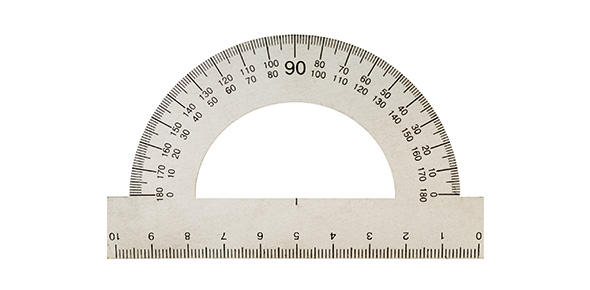# Take This Quiz On Customary And Metric Measurement!

10 Questions | Attempts: 836
ShareSettingsThere are two basic systems of measurement one is customary and another is the metric system. In the United States, the customary system of measurement is followed, whereas the metric system or SI was first adopted in France. This quiz is designed to test students knowledge of measurement, Standard and Metric. So, let's try out the quiz. All the best!

• 1.
A centimetre is what decimal of a meter?
• A.

One tenth

• B.

One hundredth

• C.

One thousandth

• D.

None

• 2.
Two grams equals how many kilograms?
• A.

2000 kg

• B.

.002 kg

• C.

2.000 kg

• D.

0.002 kg

• 3.
Which of the following is NOT a unit of measurement?
• A.

Temperature

• B.

Length and area

• C.

Mass

• D.

None of the above

• 4.
Temperature is one unit of measurement. Which tool is used to measure temperature?
• A.

Scale

• B.

Thermometer

• C.

Pound

• D.

Hammer

• 5.
This tool can be used to measure length and area. Check all that apply.
• A.

Meterstick

• B.

ruler

• C.

Thermometer

• D.

None of the above

• 6.
True or False? There are two systems of measurement used in the U.S. - the English system and the metric system.
• A.

True

• B.

False

• 7.
What is the metric system based on?
• A.

Temperature

• B.

Pounds

• C.

meter and kilogram

• D.

Kilogram

• 8.
A ______________ measures the weight of a pumpkin
• A.

Pound

• B.

Scale

• C.

Option 3

• D.

Option 4

• 9.
Water freezes at 0 degrees Celsius and boils at 100 degrees Celsius. Which system is used to report this measurement?
• A.

Metric Units

• B.

English Units

• 10.
What customary system units would you use to measure the speed of a moving object?
• A.

Kilometers

• B.

Meters

• C.

Miles

• D.

Centimetres

## Related TopicsBack to top
×

Wait!
Here's an interesting quiz for you.